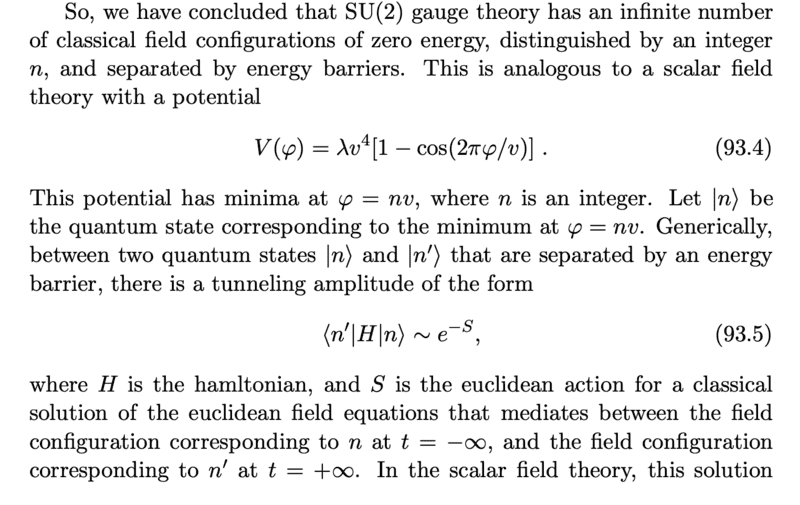# Metastable vacuum and tunneling

paralleltransport
TL;DR Summary
Hi all,

I'm currently reading about instantons and theta vacua (section 93, p 572 of http://web.physics.ucsb.edu/~mark/ms-qft-DRAFT.pdf)

Srednicki remarks in passing the following:What is a good way to "see" 93.5 is true? Is there a slightly simpler way than below which is my current understanding (given srednicki just says this i assume there must be a simple intuition behind it)

For a a set of 2 classically degenerate minimum, the energy splitting between them can be computed 2 ways:
1. One way is to compute n′|H|m. Degenerate perturbation theory says that the energy split will be proportional to this value.
2. The other method is to compute the saddle point expansion of the euclidean path integral. In this expansion one has to sum over all saddles. one of the saddle is a classical trajectory from vacuum n -> n' in an inverted potential. The energy of the true vacuum can be computed by taking the large time limit and the ln(Z) which will have an e^{S} term}2.